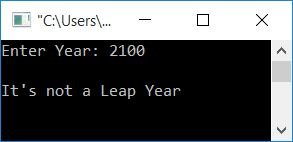Leap year program in C

# Leap year program in C

In this article, you will learn and get code about checking any given year is a leap year or not. Before going to the program, here is the formula to check whether the given year is a leap year or not.

• Year that is divisible by 4 but not divisible by 100
• Or, Year that is divisible by 400

To understand, from where this formula came, then refer to Leap Year Formula Explained. Now let's move on and implement it in a C program.

## Leap Year Program in C

Now I'm sure that you have complete understanding about what the leap year is and how it gets calculated. So its time to apply it in program:

```#include<stdio.h>
#include<conio.h>
int main()
{
int year;
printf("Enter Year: ");
scanf("%d", &year);
if((year%4==0) && (year%100!=0))
printf("\nIt's a Leap Year");
else if(year%400==0)
printf("\nIt's a Leap Year");
else
printf("\nIt's not a Leap Year");
getch();
return 0;
}```

This program was compiled and executed using Code::Blocks IDE. Here is the first snapshot of sample run:Supply any year say 2100 as input and press ENTER key to see the output as shown in the snapshot given below:It is a very simple program, you can easily understand it as all the logic used behind leap year is described in the early of this article. So let's move on the another program using function.

### Check Leap Year or Not in C using Function

This program continue checking for leap year, until user wants to terminate it. Because here, we have added some extra code, so that user becomes able to check leap year for any number time at one run only. To check leap year, this program uses function. Let's have a look at it:

```#include<stdio.h>
#include<conio.h>
int checkLeapFun(int);
int main()
{
int year, lORn;
char choice='y';
while(choice=='y')
{
printf("Enter Year: ");
scanf("%d", &year);
lORn = checkLeapFun(year);
if(lORn == 0)
printf("It's a Leap Year");
else
printf("It's not a Leap Year");
printf("\n\nWant to check more ? (y/n): ");
scanf(" %c", &choice);
printf("\n");
}
getch();
return 0;
}
int checkLeapFun(int yr)
{
if((yr%4==0) && (yr%100!=0))
return 0;
else if(yr%400==0)
return 0;
else
return 1;```